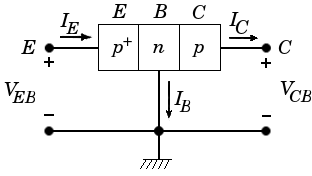## Bipolar pnp Transistorcommon base configuration

To calculate the Collector current IC in this circuit:Which can be rewritten to get VBE:Now VBE can be inserted in the following formula and we get IC:IC [mA] -5 -4 -3 -2 -1 0 1 2 3 4 5 0.00 0.05 0.10 0.15 0.20 0.25 0.30 VBC [V]
 αF = αR = IES = A ICS = A UBC(max) = V T = K IE = mA IE = mA IE = mA IE = mA IE = mA IE = mA IE = mA IE = mA IE = mA IE = mA

[note: values in textfields can be increased and decreased by Arrow-Up and Arrow-down]